# Calculate Percent of Total

info

Use AQL to do this simpler and faster: Percent of Total

Percent of Total enables you to find the percent distribution of a measure, broken down by one or many dimensions. In this guide, I will walk you through the detailed steps on how to find the percentage of a value in the total with Holistics modeling.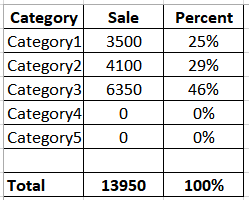## Context​

Let's say we have a table/model called percentage_of_total_raw that contains 6 fields: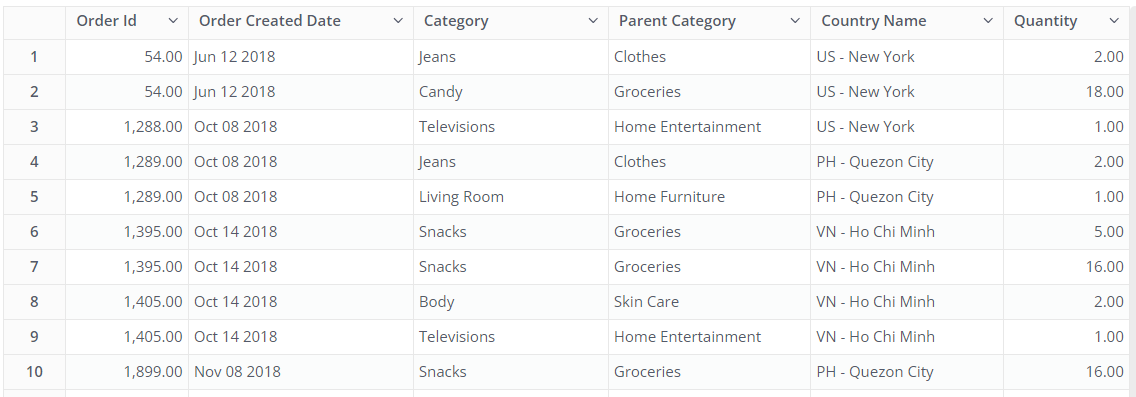Table orders {  field order_id integer  field order_created_date datetime  field category varchar  field parent_category varchar  field country_name varchar  field quantity integer}

Let us assume we want to build 2 reports that can:

• Find out the sales percentage of each category with respect to the total sales (based on quantity).
• Find out the percent of total sales per category and country for each individual day. This will affect the denominator of the percentage that the total sales are grouped by each day.
• Filter the report by any dimension (category, date...) and when using a filter, percent of total does not reflect the percent of filtered records to the total records in the data.

This is how the 1st report would finally appear: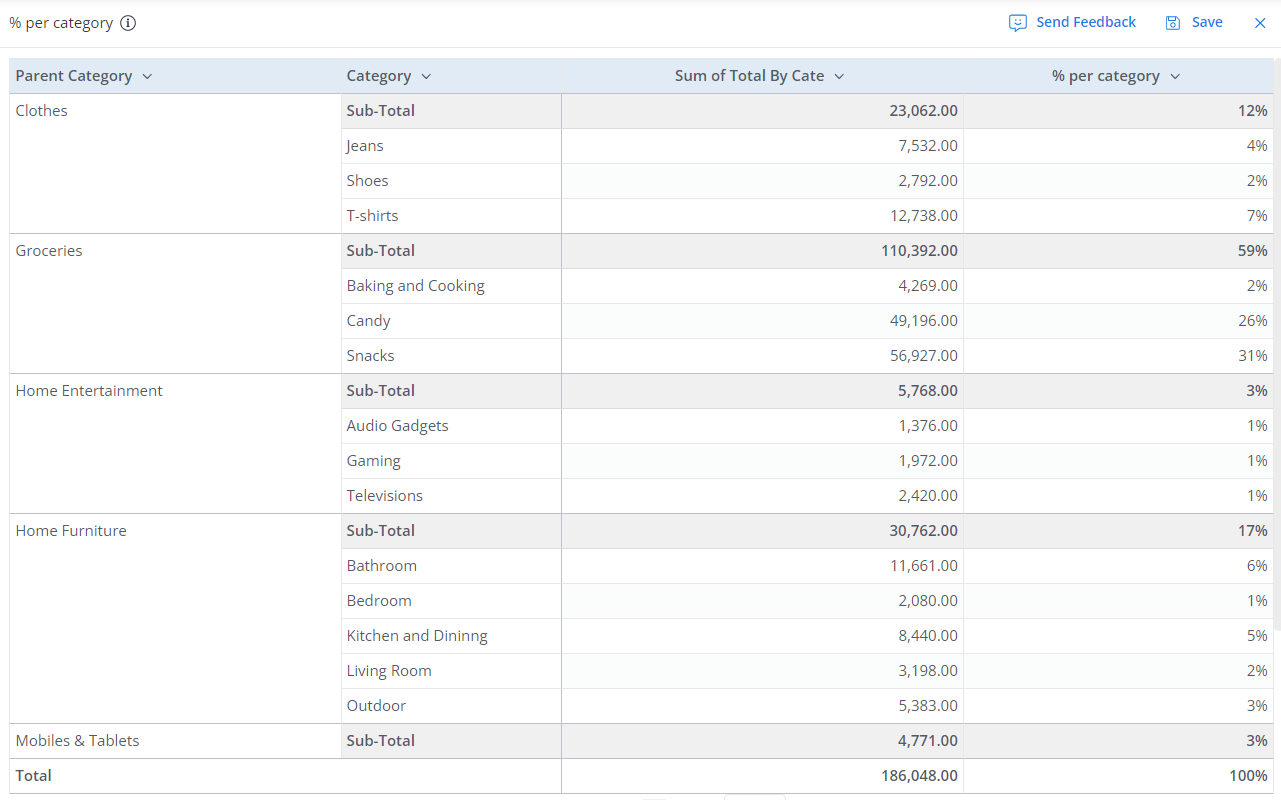## General Solution​

$\large percentage = {Partial\space Amount \over Total\space Amount}$

We perform some SQL transformations to enable granular calculations of the percentage of total with the following steps:

• Create Model 1 or Subquery 1: Calculate the numerator of the percentage in which the total value is grouped by one or many dimensions.
• Create Model 2 or Subquery 2: Determine the whole or total amount of what you want to find a percentage for in the denominator of the percentage.
• Link these models or join these subqueries and add them to the exploration.
• And finally, you need to divide the sub amount by the total using a business calculation to get the percentage.

## Use case 1: The simple percent of total sales per category​

In this case, we would like to divide the total sum by only one attribute such as category.

Particularly, we need to take the Sum of sale quantities for each category and divide it by the Total sale quantities for all categories.

$\large percentage = { 𝐒𝐮𝐦\space(quantity)\space \space 𝐠𝐫𝐨𝐮𝐩\space 𝐛𝐲\space(category,country,date) \over 𝐒𝐮𝐦\space(quantity)\space \space 𝐠𝐫𝐨𝐮𝐩\space 𝐛𝐲\space(date)}$

### Using transform model​

1. First, we build the percent_of_total_per_category (category, total_by_cate, total) model where total_by_cate measures how many quantities are in each category, and total is the total sales of all categories in the denominator.

We do that by creating two subqueries to calculate the numerator total_by_cate and the denominator total and then, join them ON TRUE

with pt_all as (  select    sum(quantity) as total  from    {{ #percentage_of_total_raw t1}}    ) -- to calculate denominator (total),pt_sale_by_cate as (  select    category,    sum(quantity) as total_by_cate  from    {{ #percentage_of_total_raw }}  group by 1    ) -- to calculate numerator (total_by_cate)select  pt_sale_by_cate.category,  pt_sale_by_cate.total_by_cate ,  pt_all.totalfrom pt_all join pt_sale_by_cate on true
2. Put the data model in a data set and calculate percentage with business calculation

To get the percent of total per category, we will create a new measure called % per category which divides total_by_cate by total

sum(total_by_cate) / max(total)

### Building the chart​

#### Using Pivot Table​

Drag your category to "Rows" and percentage_of_total_raw.quantity to "Values" with setting to Sum.

Add the calculation % per category to "Values".

Go to "Styles" to enable your Column Totals and Sub Total. Now you have all your percent of total per category as the out put we showned in Context part.

#### Using Pie/Donut Chart​

To display a set of categories’ proportions or percentages of the total per one attribute, you can just simply use a pie chart or donut chart. There is no need to use the transform model to first work out the percentage of the pie chart that each category should occupy. You can just let the visualization do the percent of total calculation by itself.

The percentages will be listed in the legend, alongside the records to which they belong. Under "Styles", enable "Show percentage" to display the percentage that the sectors represent instead of the raw value.Note: A pie chart is often used to compare each group’s contribution to the whole, as opposed to comparing groups to each other.

## Use case 2: The percent of total per category and country for each day​

The result will show:

• The order's country and category
• Total orders (sale quantities) of each category for each certain day
• Percentage of that category over total sales of all categories for each certain day

$\large percentage = { 𝐒𝐮𝐦\space(quantity)\space \space 𝐠𝐫𝐨𝐮𝐩\space 𝐛𝐲\space(category) \over 𝐒𝐮𝐦\space(quantity)}$

### Overall data transformation​

Using the base model percentage_of_total_raw, you can simply measure the numerator using aggregate function SUM in the exploration UI.

You will need an orders_total_not_broke_down_by_category model to calculate the total sales of all categories on each day in the denominator.

In order to get the single entry point for 2 models, you need a date dimension model (date_dim model) and join it with percentage_of_total_raw and orders_total_not_broke_down_by_category using a created common date field in all 3 models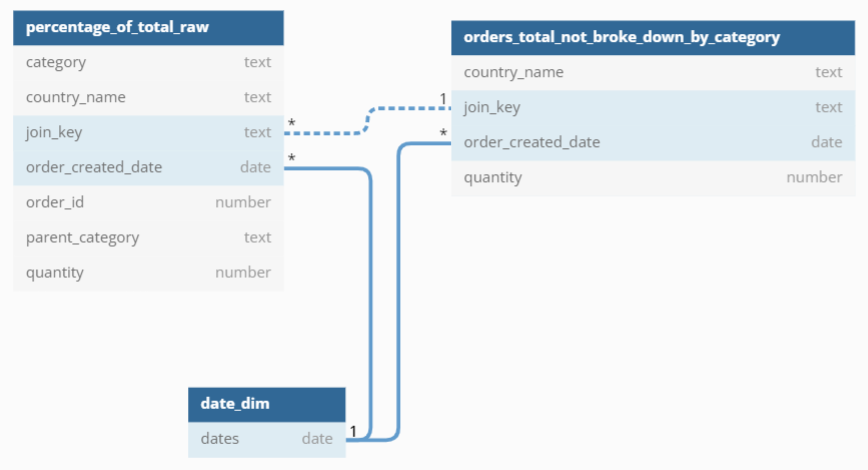Note: In this case, the denominator number is the total sum grouped by each date. That's why we chose date_dim model as a junction model. In other quarters, we use date_dim model to compare metrics from different models by date.

### Create Orders Total Not Broken Down By Category model​

select    {{ #t1.order_created_date }},    {{ #t1.country_name }},    -- **We remove the category column here** {{ #t1.category }},    sum({{ #t1.quantity }}) as quantityfrom {{ #percentage_of_total_raw t1}}group by 1,2

### Joining the models​

To add the orders_total_not_broke_down_by_category model to the same exploration with percentage_of_total_raw, we have to link them somehow.

An experienced modeler might notice that we can create a ‘1 - n’ relationship from orders_total_not_broke_down_by_category to percentage_of_total_raw by creating a join_key to both the original model and the total model like country_name || '-' || CAST(date as text). The problem with that approach is that only the total from countries that exist in a category would show up.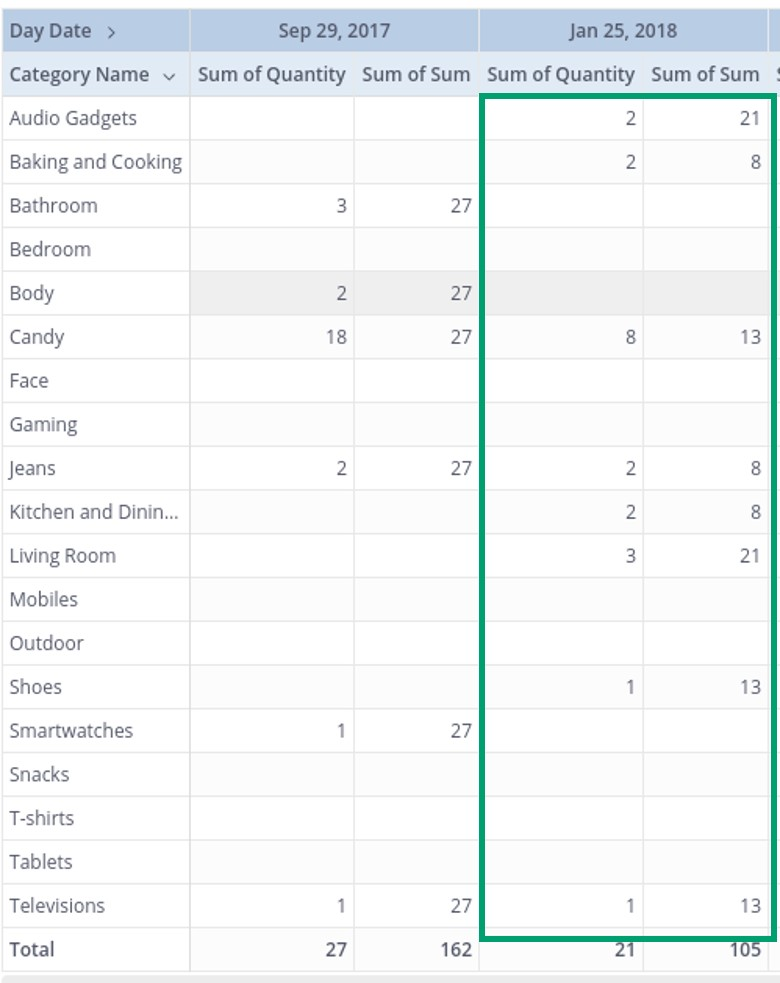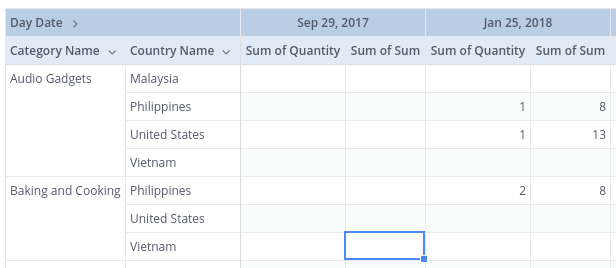The recommended way to deal with this is to create a [date_dim model](https://docs.holistics.io/guides/using-date-dim-model).

### Create Date Dims model​

date_dim - a created common model to be the base of JOIN operations

select  generate_series( min({{ #a.order_created_date }}), max({{ #a.order_created_date }}),'1d')::date as datesfrom  {{ #percentage_of_total_raw a}}

Date in date_dim model is unique so we can create two ‘1 - n’ relationships to percentage_of_total_raw and orders_total_not_broke_down_by_category .

Now orders_total_not_broke_down_by_category returns the total for the day regardless of category.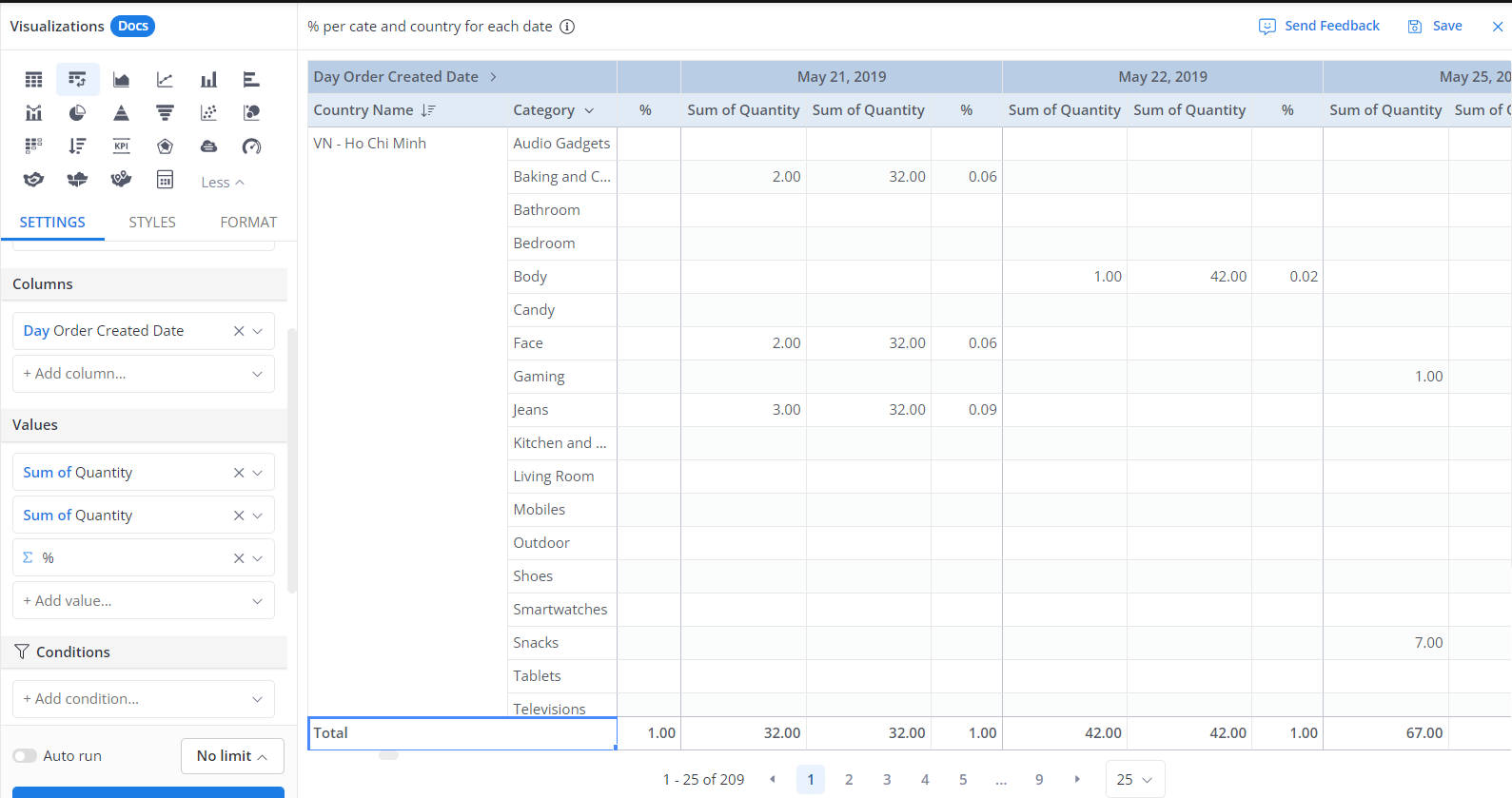### Create the dataset and calculate the percentage​

Now, create the dataset, add the orders_total_not_broke_down_by_category, percentage_of_total_raw and date_dim model to the dataset and it will be ready to be explored.

Calculate percentage % for each day with business calculation as below:

sum(percentage_of_total_raw.quantity) / sum(orders_total_not_broke_down_by_category.quantity)

## Building the chart​

When you only need the percent of the total in the visualization, you can use one of the following chart types to do the percent of total calculation:

• Column
• Bar
• Scatter chart
• Line
• Area
• Pie chart and Donut chart

When you need both the partial amount and the percent of the total in the visualization, you can use:

• Table and Pivot Table
• Combination Chart.

### Displaying using Column chart​

Here is an example to show how the chart looks and acts using Column chart with "Stack Series" option.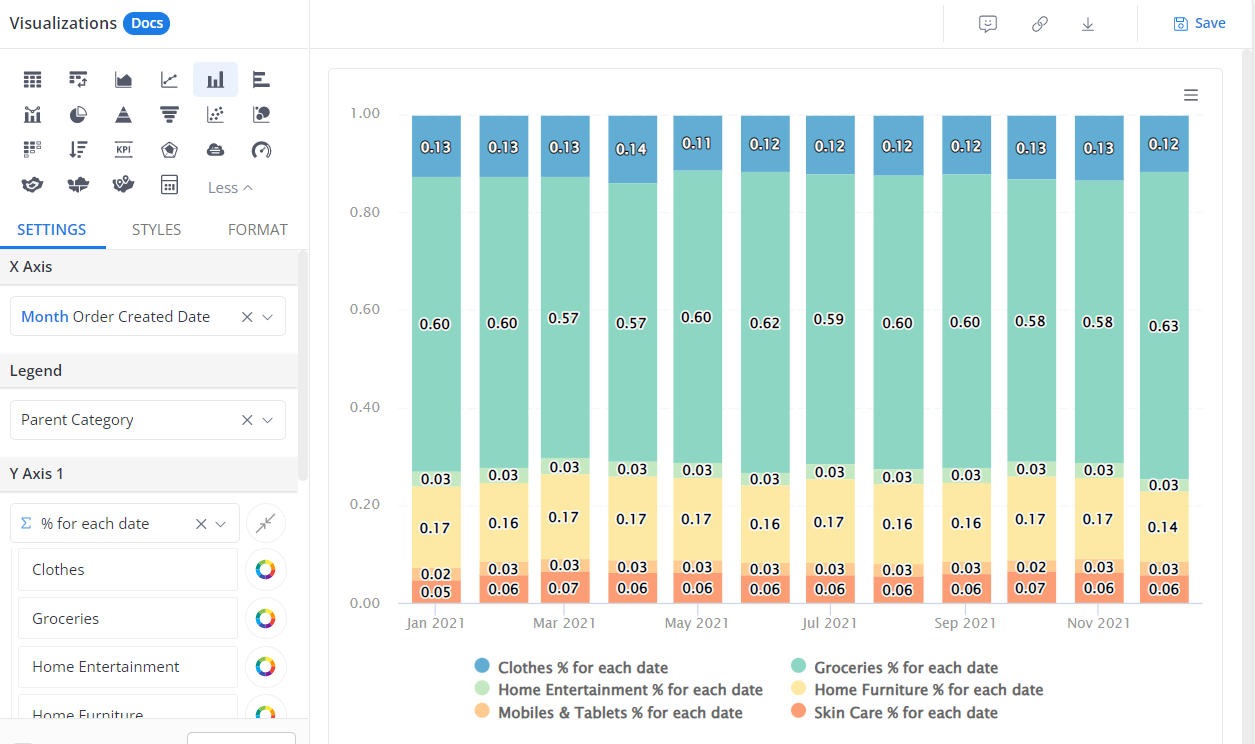### Displaying using Pivot Tables​

Drag your  category to "Rows", Day order_created_date to "Columns" and percentage_of_total_raw.quantity , orders_total_not_broke_down_by_category.quantity to "Values" set to Sum.

Add the calculation % for each day to "Values".

Go to "Styles" to enable your Column Totals and Sub Total. Now you have all your percent of total per category for each day.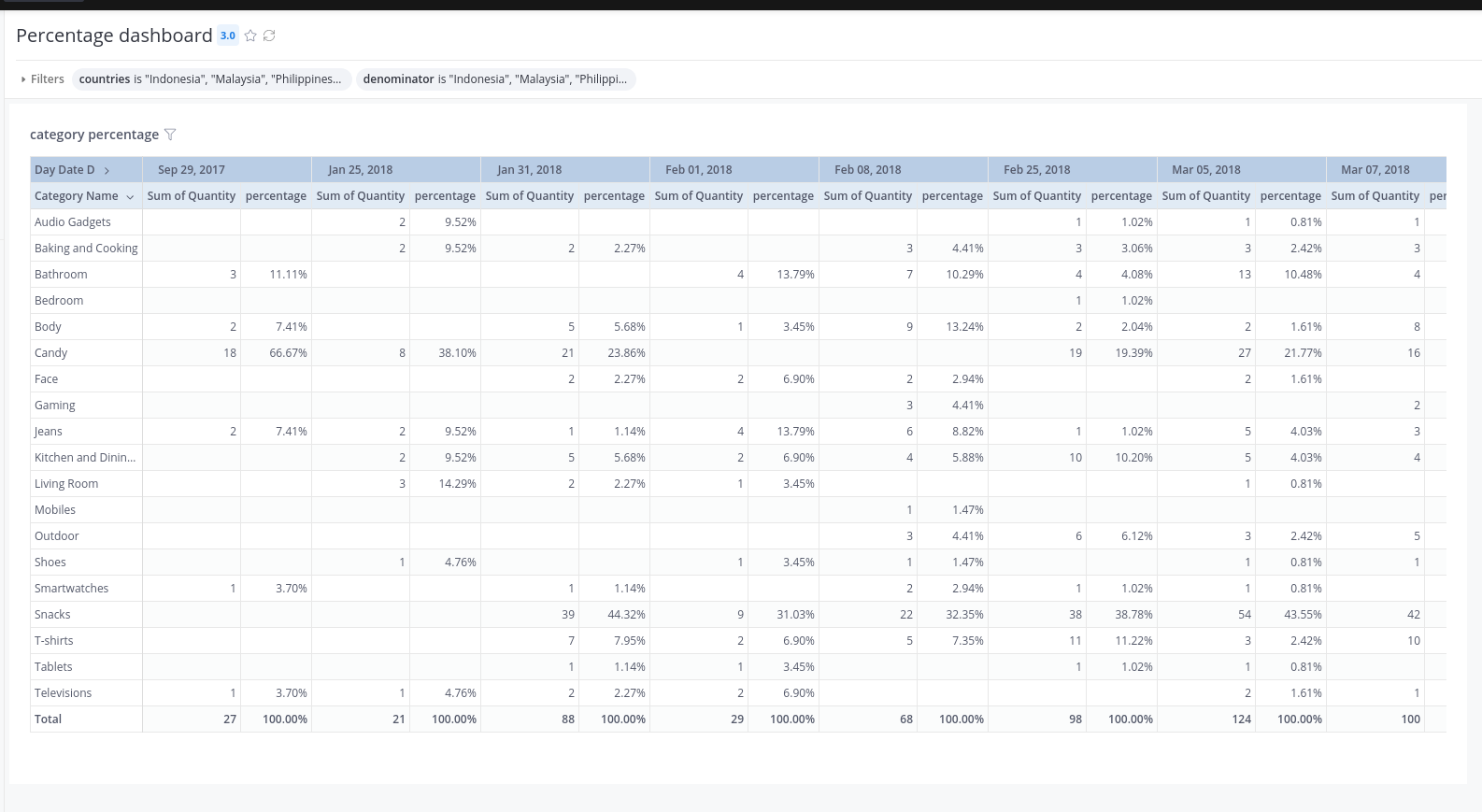### Dashboard filters​

Right now, the filter can only be mapped to one field in one data model of the dataset so you'll have to create two filters, one to filter the numerator, one to filter the denominator.

## Other Notes​

### Percentage options​

Computing a percentage involves specifying a total on which the percentage is based. With this transform model, you can choose many different options: based on the entire table, a column, and a row.

For example, in use case 2, each measure on the table is expressed as a percentage of the total for the column. The values within the "percentage" column add up to 100%. Based on the ways that you build the transform models and link them, you can also set each measure on the worksheet so that it is expressed as a percentage of the total for the row or for the entire table.

### Preserve “Percent of Total” when using dashboard filter​

If you want to filter out one or more of your dimensions, the ‘percent of total figure’ changes because the ‘total’ which is used in the denominator computation changes too to reflect the loss of the dimension members.

In some cases, you will want to keep the original percentage (of the whole underlying data) while just displaying the dimension members you are interested in. or example, we have a table for Sales per category.When we set a dashboard filter to only show Category = Category1, then the percent will be changed to 100%, as all other categories were excluded from the total.

Suppose that you want to keep the original percent of Category1 (25%).

What you can do is set up a filter that only filters the numerator. So the % of the total calculation will not change when you adjust the filter. This is due to the ‘total’ computed in the denominator isn't being changed.